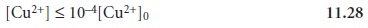Home | | Modern Analytical Chemistry | Controlled-Potential Coulometry

# Controlled-Potential Coulometry

The easiest method for ensuring 100% current efficiency is to maintain the working electrode at a constant potential that allows for the analyte’s quantitative oxidation or reduction, without simultaneously oxidizing or reducing an interfering species.

Controlled-Potential Coulometry

The easiest method for ensuring 100% current efficiency is to maintain the working electrode at a constant potential that allows for the analyte’s quantitative oxidation or reduction, without simultaneously oxidizing or reducing an interfering species. The current flowing through an electrochemical cell under a constant potential is proportional to the analyte’s concentration. As electrolysis progresses the analyte’s concentration decreases, as does the current. The resulting current-versus-time pro- file for controlled-potential coulometry, which also is known as potentiostatic coulometry, is shown in Figure 11.20. Integrating the area under the curve (equa- tion 11.25), from t = 0 until t = te, gives the total charge. In this section we consider the experimental parameters and instrumentation needed to develop a controlled- potential coulometric method of analysis.## Selecting a Constant Potential

In controlled-potential coulometry, the potential is selected so that the desired oxidation or reduction reaction goes to completion without interference from redox reactions involving other components of the sam- ple matrix. To see how an appropriate potential for the working electrode is se- lected, let’s develop a constant-potential coulometric method for Cu2+ based on its reduction to copper metal at a Pt cathode working electrode.

Cu2+(aq)+ 2e < = = = = > Cu(s)   ………………….. 11.26

A ladder diagram for a solution of Cu2+ (Figure 11.21) provides a useful means for evaluating the solution’s redox properties. From the ladder diagram we can see that reaction 11.26 is favored when the working electrode’s potential is more negative than +0.342 V versus the SHE (+0.093 V versus the SCE). To maintain a 100% cur- rent efficiency, however, the potential must be selected so that the reduction of H3O+ to H2 does not contribute significantly to the total charge passed at the electrode.The potential needed for a quantitative reduction of Cu2+ can be calculated using the Nernst equation11.27where [Cu2+]0 is the initial concentration of Cu2+ in the sample. Substituting equa- tion 11.28 into equation 11.27 gives the desired potential electrode asIf the initial concentration of Cu2+ is 1.00 x 10–4 M, for example, then the cathode’s potential must be more negative than +0.105 V versus the SHE (–0.139 V versus the SCE) to achieve a quantitative reduction of Cu2+ to Cu. Note that at this potential H3O+ is not reduced to H2, maintaining a 100% current efficiency. Many of the published procedures for the controlled-potential coulometric analysis of Cu2+ call for potentials that are more negative than that shown for the reduction of H3O+ in Figure 11.21.12 Such potentials can be used, however, because the slow kinetics for reducing H3O+ results in a significant overpotential that shifts the potential of the H3O+/H2 redox couple to more negative potentials.

## Minimizing Electrolysis Time

The current-time curve for controlled-potential coulometry in Figure 11.20 shows that the current decreases continuously throughout electrolysis. An exhaustive electrolysis, therefore, may require a long time. Since time is an important consideration in choosing and designing analyt- ical methods, the factors that determine the analysis time need to be considered.

The change in current as a function of time in controlled-potential coulometry is approximated by an exponential decay; thus, the current at time t is

i = i0ekt   ………………….. 11.29

where i0 is the initial current, and k is a constant that is directly proportional to the area of the working electrode and the rate of stirring and inversely proportional to the volume of the solution. For an exhaustive electrolysis in which 99.99% of the analyte is oxidized or reduced, the current at the end of the analysis, te, may be ap- proximated as

i  (10–4)i0   ………………….. 11.30

Substituting equation 11.30 into equation 11.29 and solving for te gives the mini- mum time for an exhaustive electrolysis asFrom this equation we see that increasing k leads to a shorter analysis time. For this reason controlled-potential coulometry is carried out in small-volume electrochem- ical cells, using electrodes with large surface areas and with high stirring rates. A quantitative electrolysis typically requires approximately 30–60 min, although shorter or longer times are possible.

## Instrumentation

The potential in controlled-potential coulometry is set using a three-electrode potentiostat. Two types of working electrodes are commonly used: a Pt electrode manufactured from platinum-gauze and fashioned into a cylindrical tube, and an Hg pool electrode. The large overpotential for reducing H3O+ at mercury makes it the electrode of choice for analytes requiring negative potentials. For example, potentials more negative than –1 V versus the SCE are feasible at an Hg electrode (but not at a Pt electrode), even in very acidic solu- tions. The ease with which mercury is oxidized, however, prevents its use at po- tentials that are positive with respect to the SHE. Platinum working electrodes are used when positive potentials are required. The auxiliary electrode, which is often a Pt wire, is separated by a salt bridge from the solution containing the an- alyte. This is necessary to prevent electrolysis products generated at the auxiliary electrode from reacting with the analyte and interfering in the analysis. A satu- rated calomel or Ag/AgCl electrode serves as the reference electrode.

The other essential feature of instrumentation for controlled-potential coulom- etry is a means of determining the total charge passed during electrolysis. One method is to monitor the current as a function of time and determine the area under the curve (see Figure 11.20). Modern instruments, however, use electronic integration to monitor charge as a function of time. The total charge at the end of the electrolysis then can be read directly from a digital readout or from a plot of charge versus time (Figure 11.22).Study Material, Lecturing Notes, Assignment, Reference, Wiki description explanation, brief detail
Modern Analytical Chemistry: Electrochemical Methods of Analysis : Controlled-Potential Coulometry |